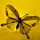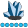## Code is not working as intended

%let x= 2020Q1;

%let y= 0;

%macro xyz();

%if &y =0 %then

%let abc = %sysfunc(intnx(qtr, %sysfunc(inputn(&x, yyq6.)),0,b),yyq6.);

%mend;

%xyz;

1 ACCEPTED SOLUTION

Accepted Solutionswhymath
Lapis Lazuli | Level 10

## Re: Code is not working as intended

If you want to use macro variable abc outside of macro, you need to make it a global macro variable:

``````%let x= 2020Q1;
%let y= 0;

%macro xyz();
%global abc;
%if &y =0 %then
%let abc = %sysfunc(intnx(qtr, %sysfunc(inputn(&x, yyq6.)),0,b),yyq6.);
%mend;

%xyz;
%put &=abc;

ABC=2020Q1``````

And you may want to know Scopes of Macro Variableswhymath
Lapis Lazuli | Level 10

## Re: Code is not working as intended

If you want to use macro variable abc outside of macro, you need to make it a global macro variable:

``````%let x= 2020Q1;
%let y= 0;

%macro xyz();
%global abc;
%if &y =0 %then
%let abc = %sysfunc(intnx(qtr, %sysfunc(inputn(&x, yyq6.)),0,b),yyq6.);
%mend;

%xyz;
%put &=abc;

ABC=2020Q1``````

And you may want to know Scopes of Macro Variables

Discussion stats